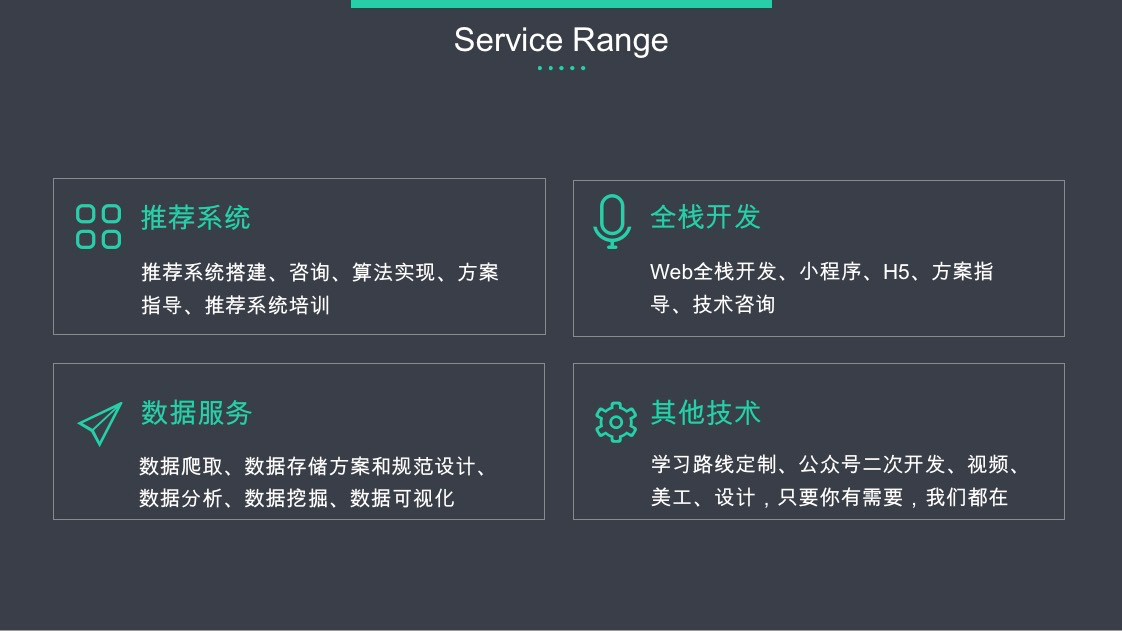• 协同过滤优化技巧中矩阵分解（MF）的限制
• 如何基于神经网络优化协同过滤

# 背景

• 提出了一种神经网络架构来对用户和物品的潜在特征进行建模，并为基于神经网络的协同过滤设计了通用的框架NCF
• 证明了MF可以被解释为NCF的一个特例，并利用多层感知器增加NCF模型的高度非线性。
• 基于两个真实的数据集证实了NCF的有效性

# 基础知识

## 2、矩阵分解（MF）

MF将每个用户和物品的联系用潜在的向量特征来表示，用$p_u$和$q_i$分别表示用户和物品的隐含的向量，MF的计算方式是 $p_u、q_i$的内积，如下所示：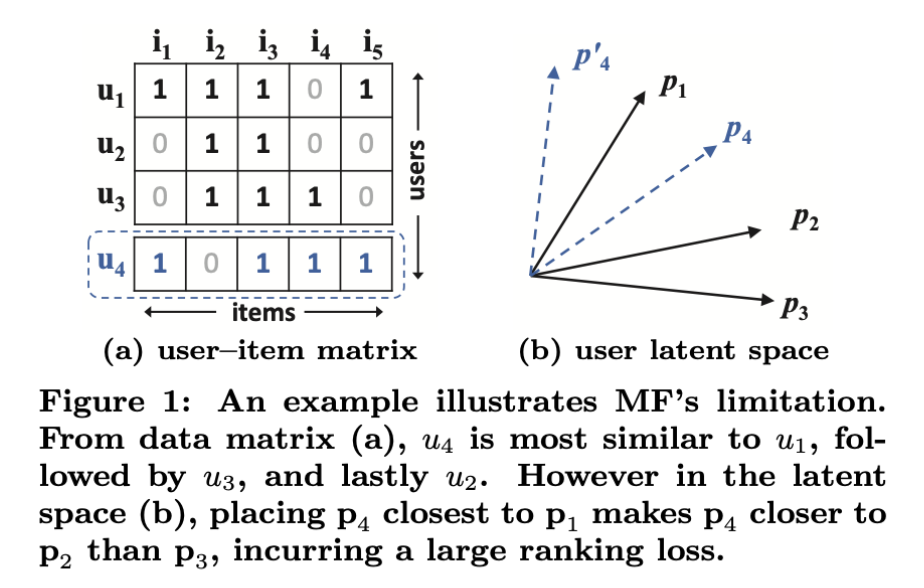• 使用内积方式或者余弦相似度进行计算时，用户和物品的隐含向量特征维度必须一致
• 使用jaccard相似计算修正计算两个用户的相似度

# 神经网络协同过滤

## 1、NCF框架

### a. 框架介绍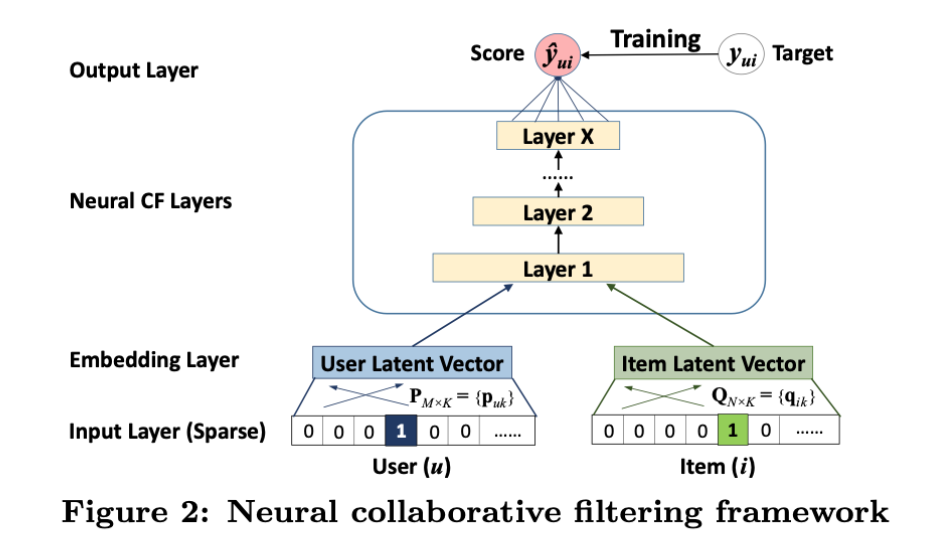Input Layer输入的是稀疏向量，经过Embedding Layer转转化为稠密向量，之后向量被传入到多层的神经网络，这一整块被称为 Neural CF Layers，最后一层称为 Output Layer，输出层的维度决定了模型的功能，输出的$\hat{y}_{ui}$为预测分值，模型训练的目标是最小化$\hat{y}_{ui}、y_{ui}$之间的误差，基于point wise方式的损失函数进行优化（关于point wise、pair wise、list wise的区别可以参考： LTR｜怎么理解基于机器学习“四大支柱”划分的学习排序方法）。

• $P, Q$表示用户和物品的隐向量
• $\Theta_f$表示函数$f$的参数

• $\phi_{out}$ 表示输出层
• $\phi_x$ 表示第$x$层，总共有$X$层

### b. 参数学习

• $Y$ 表示正样本
• $Y^-$ 表示负样本（可以是全部负样本，也可以是抽样后的）

## 2、GMF（Generalized Matrix Factorization）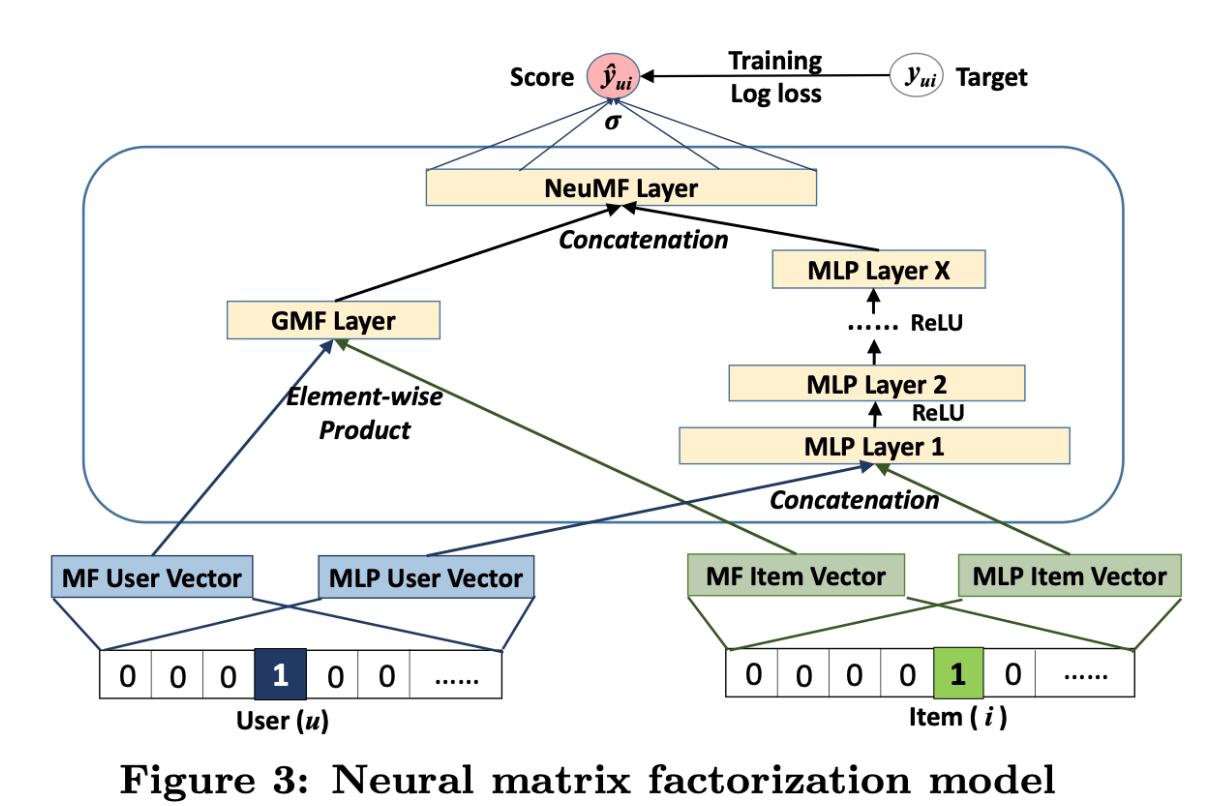GMF对应Figure 3 中的左半部分，用$p_u$表示用户的隐向量，用$q_i$表示物品的隐向量，可以定义GMF的函数为：

$\odot$表示内积计算。将结果传到输出层，对应的计算公式为：

• $a_{out}$ 表示激活函数，本论文中用的是$\sigma (x) = 1 \ (1 + e^{-x})$
• $h$ 表示权重向量，使用 上文中的 log loss进行学习

## 3、MLP（Multi-Layer Perception）

MLP模型的激活函数选用的ReLU（还有sigmoid，tanh可以供选择），原因是：

• sigmoid 函数在横坐标接近正负无穷时，输出值接近 1或0，会导致神经元停止学习
• tanh 函数可以有sigmoid转化得到（$tanh(x/2) = 2\sigma (x) -1$）,会造成同样的问题
• ReLU更符合生物学，且两端不饱和，同时ReLU非常适合稀疏函数，不会导致过拟合。

## 4、NeuMF（Neural Matrix Factorization）

### a. NeuMF介绍

NeuMF则是GMF和MLP的结合，整体模型的思路如Figure 3所示，整体模型的表达式为：

MLP层采用的是ReLU激活函数。

# 实验

• 基于NCF框架的方法是否优于最新的隐式协同过滤方法？
• 论文中提出的优化框架（带有负采样的对数损失）如何应用于推荐任务？
• MLP多层神经网络是否有利于用户和物品之间的行为交互？

• MovieLens
• Pinterest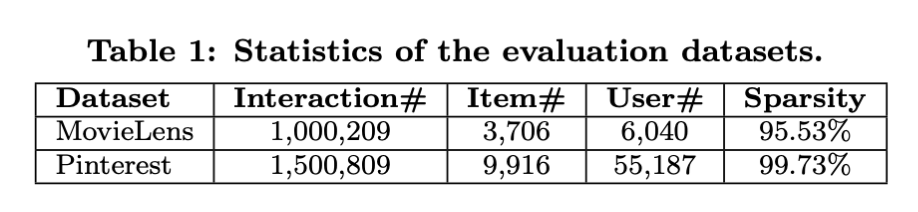• 训练集和测试集的拆分，按照时间的顺序进行拆分，最接近当前时间的为测试集
• 随机选取训练集中用户没有行为的100个物品作为测试集中的物品，因为整体的物品太多，测试过程消耗资源和时间，不利于实验
• 每个评估指标取top 10物品进行评测
• 实验的指标：Hit Ratio、Nor- malized Discounted Cumulative Gain (NDCG）
• 实验采用的对比模型有：ItemPop、ItemKNN、BRP、eALS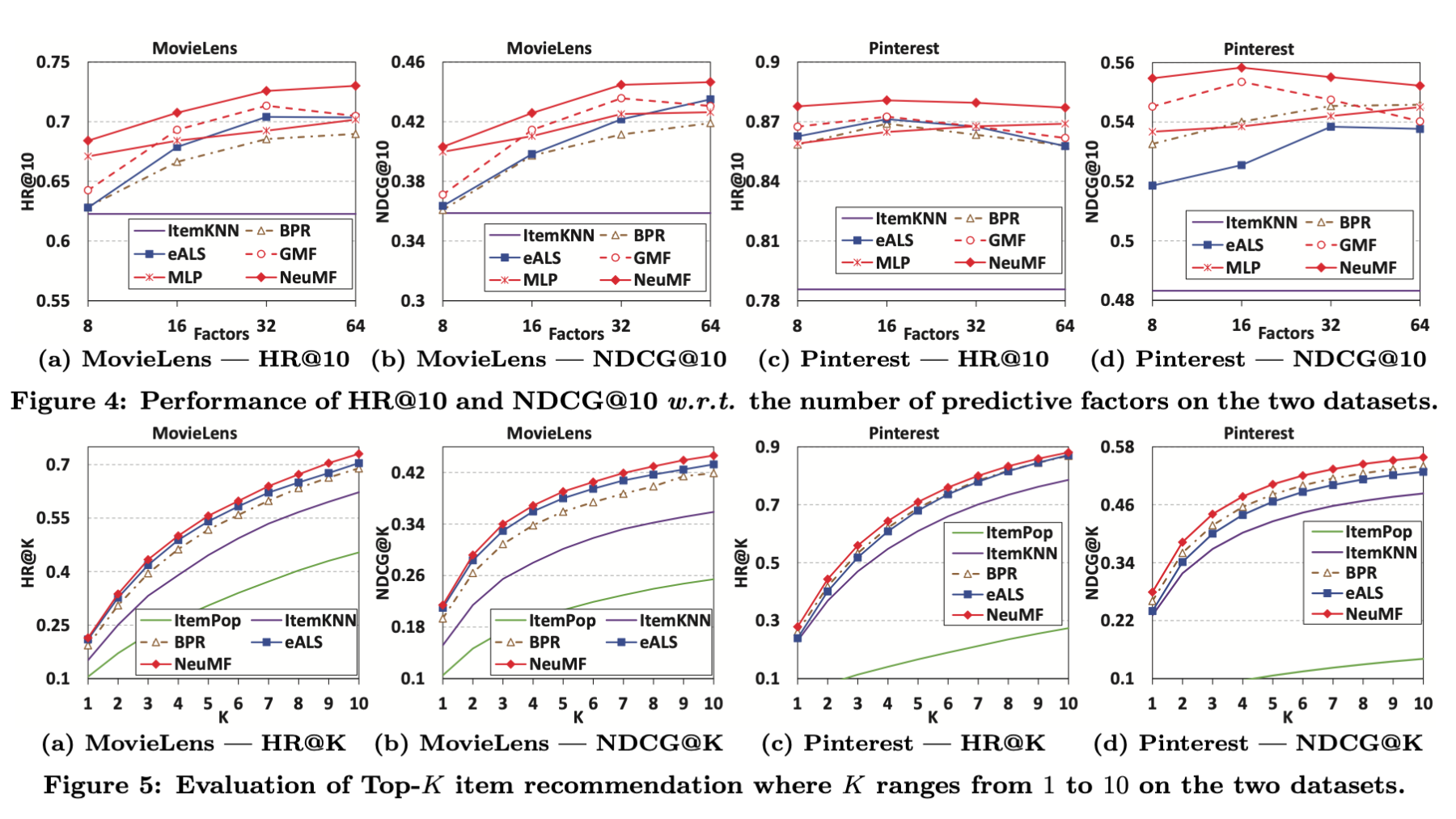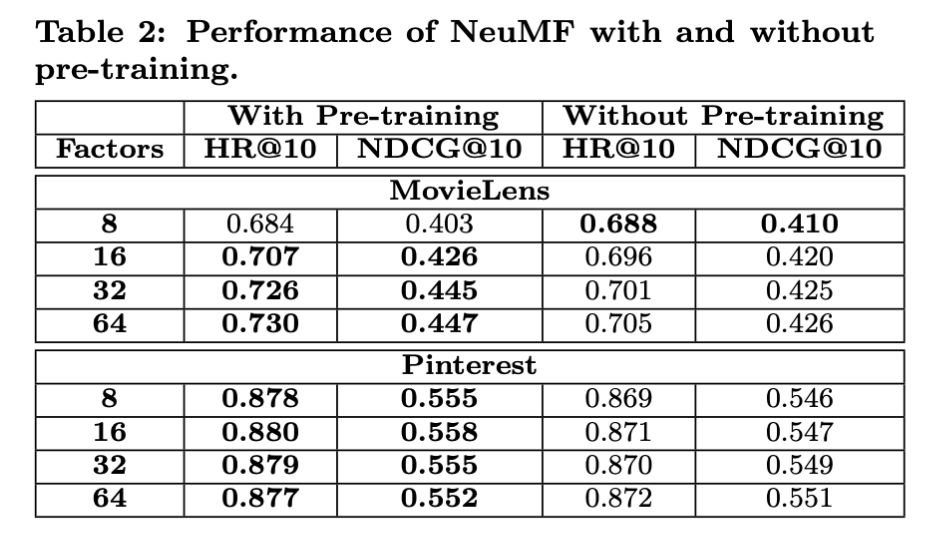、MLP、NeuMF，在这三种算法中性能对比结果为：NeuMF > MLP > GMF，如Figure 6所示。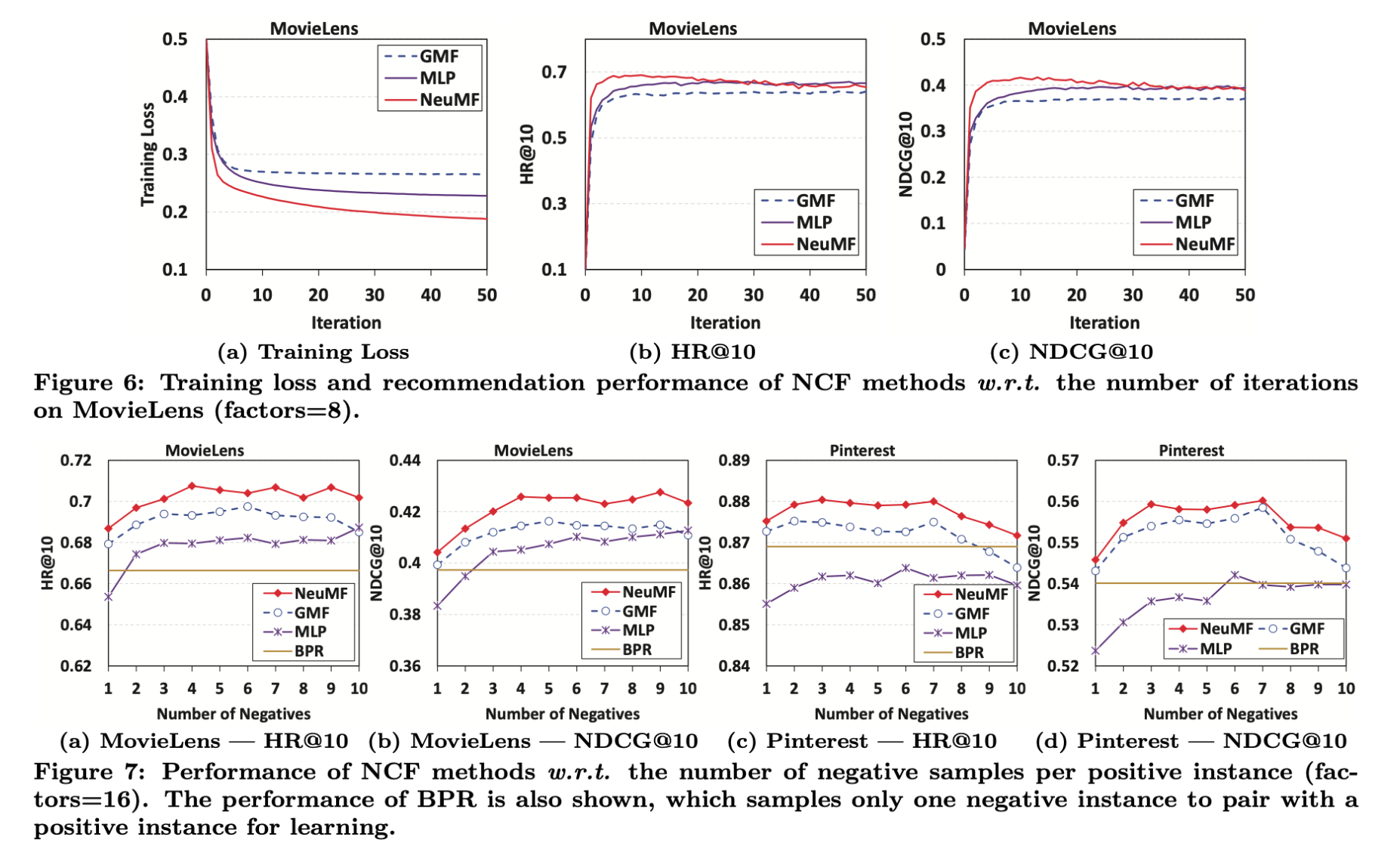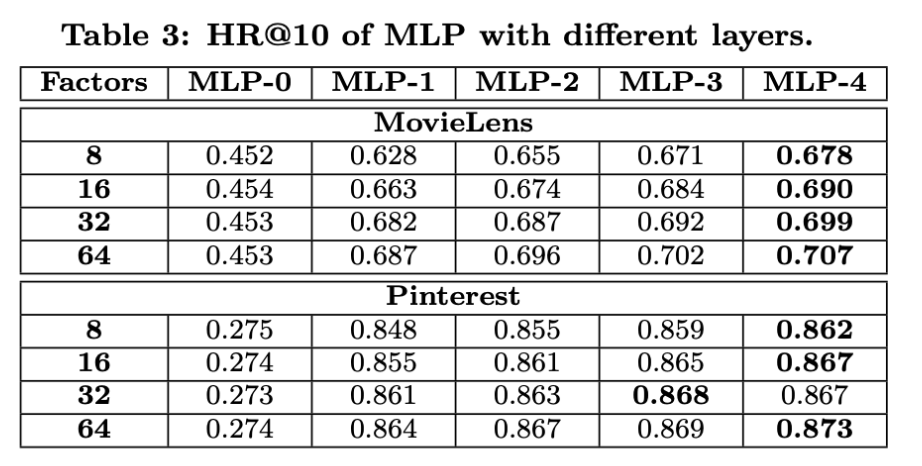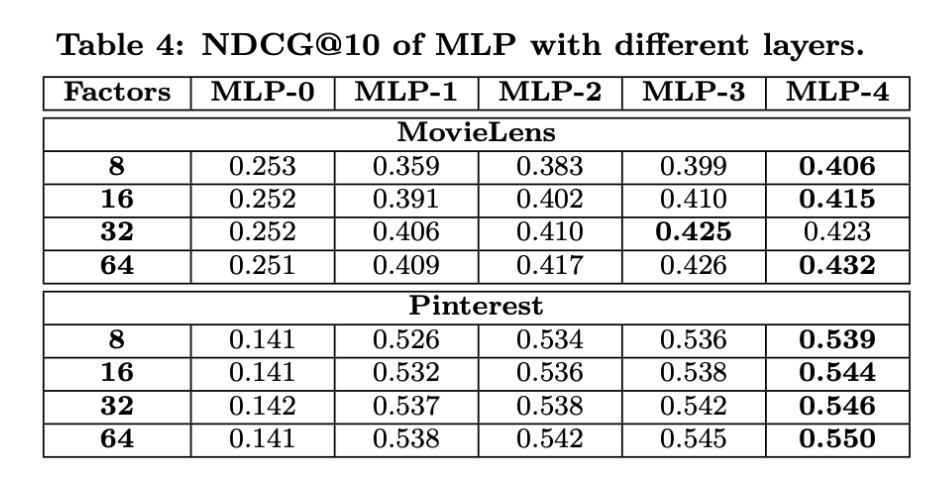【技术服务】，详情点击查看： https://mp.weixin.qq.com/s/PtX9ukKRBmazAWARprGIAg